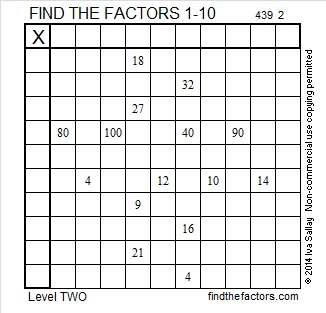# 247 and Level 2

• 247 is a composite number.
• Prime factorization: 247 = 13 x 19
• The exponents in the prime factorization are 1, and 1. Adding one to each and multiplying we get (1 + 1)(1 + 1) = 2 x 2 = 4. Therefore 247 has 4 factors.
• Factors of 247: 1, 13, 19, 247
• Factor pairs: 247 = 1 x 247 or 13 x 19
• 247 has no square factors so its square root cannot be simplified. √247 ≈ 15.716Print the puzzles or type the factors on this excel file: 10 Factors 2014-09-29